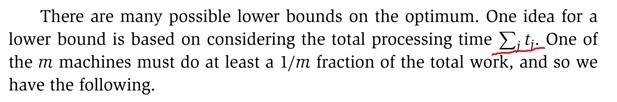# Load Balancing: Can't understand the summation term

Summary:
Hi,
I am trying to understand a summation term related to load balancing
I can’t understand the term Summation of (j tj). Are we multiplying j and tj. But the text is not talking about multiplying, it says”Total processing timeSomebody please guide me.

Zulfi.

## Answers and Replies

jedishrfu
Mentor
the summation just sums the terms.

##\sum_{n=0}^{10}{(2n+1)} = 1 + 3 + 5 + 7 + ... + 21##

in your case:

##\sum_{j=1}^{m}{(t_j)} = t_1 + t_2 + t_3 + ...##

but they left out the limits of j=1 to m.

•zak100
Hi,
Thanks for your response.

You mean its not multiplying j with ##t_j##
##\sum_j t_j ##

Thanks.

God bless you.

Zulfi.

jedishrfu
Mentor
The j is saying that is the index the summation indexes over rather like a “for”loop in programming j is the “for” loop index.